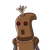# One angle of a parallelogram are 100°. Find the measure of remaining angle​

One angle of a parallelogram are 100°. Find the measure of remaining angle​

### 1 thought on “One angle of a parallelogram are 100°. Find the measure of remaining angle​”

1.Step-by-step explanation:

therefore, angle D will also be 80 degree. (opposite angles are equal)(opposite to angle B) hence, angle A = 100 degree, angle B = 80 degree, angle C = 100 degree, and, angle D = 80 degree.